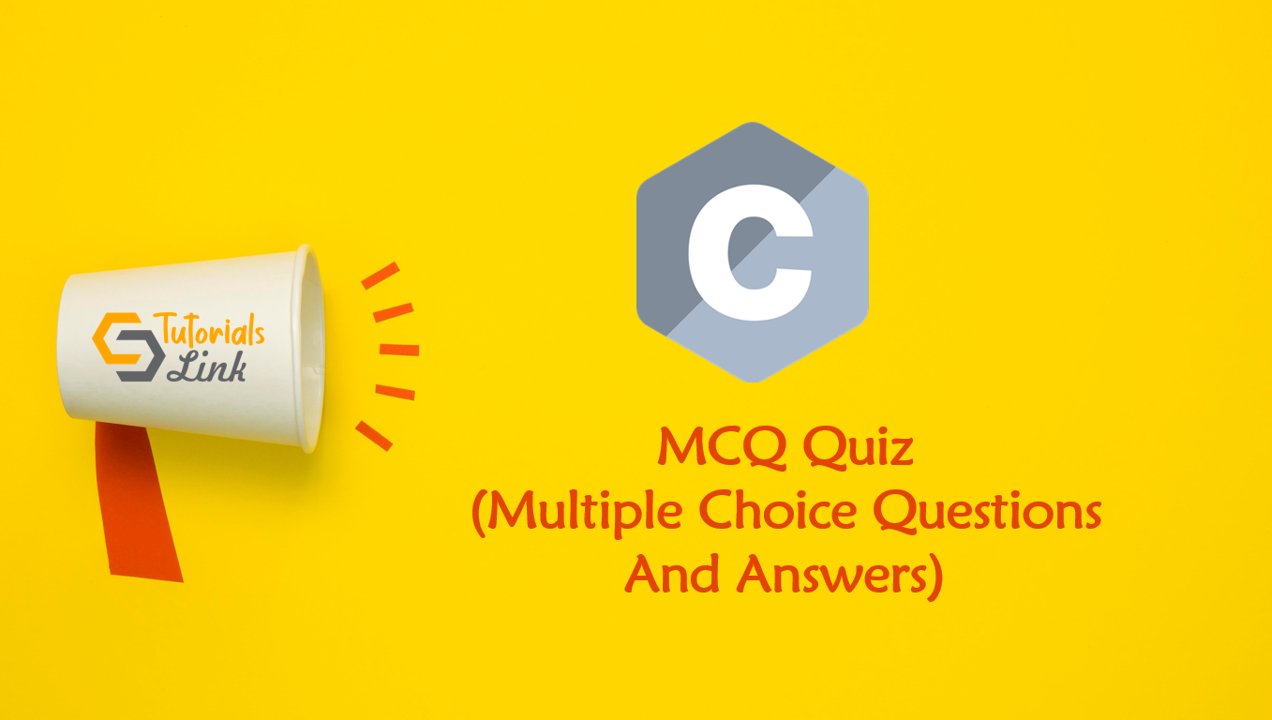Loading, please wait...# Functions in C Programming MCQ (Multiple Choice Questions And Answers)

Search here for MCQs

Are you preparing for the next job interviews? If yes, trust me this post will help you also we'll suggest you check out a big collection for Programming Full Forms that may help you in your interview:

## 26. Assume the output of the following code:

``````void myshow(int *);

void main()
{
int a=10;
printf("%d ", a);
myshow(&a);
printf("%d", a);

}

void myshow(int *k)
{
*k=20;
}``````
• 10 30
• 20 20
• 10 10
• 10 20

## 27. What will be the data type returned for the following C function?

``````#include
int func()
{
return (double)(char)5.0;
}``````
• int
• double
• char
• No output

## 28. It is not advisable to use macros instead of functions because

• it increases the code size
• no type checking will be done
• recursion is not possible
• All of the above

## 29. Assume the output of the following code:

``````void main()
{
static int x;
if (x++ < 2)
main();
}``````
• No output
• main() is called twice
• Run Time Error
• Varies

## 30. Guess the output of the following code:

``````int x = 5;
void main()
{
int x = 3;
printf("%d", x);
{
x = 4;
}
printf("%d", x);
}``````
• 3 3
• 4 4
• 3 4
• Runtime error